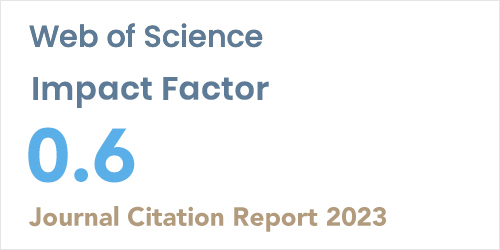ISSN: 1304-7191 | E-ISSN: 1304-7205
Volume: 41 Issue: 3 Year: 2006Numerical approach of fisher's equation with strang splitting technique using finite element galerkin method
1Department of Mathematics, Ağrı İbrahim Çeçen University, Ağrı, 04100, Turkiye
Sigma J Eng Nat Sci 2023; 41(2): 344-355 DOI: 10.14744/sigma.2023.00041

#### Abstract

In the present paper, non-linear Fisher’s reaction-diffusion equation is solved numerically by using Strang splitting technique with the help of Galerkin method combined with quadratic B-spline base functions. For this aim, Fisher’s equation is split into two sub-equations with respect to time such that one is linear and the other one is nonlinear. Galerkin method using quadratic B-spline finite elements is applied to each sub-equation. To check the correctness and reliability of the presented approach, we have realized on three numerical examples and have made a comparison with earlier studies existing in the literature calculating the error norms norms 𝐿2 𝑎𝑛𝑑 𝐿∞ .The solutions obtained in the article show that the present method is quite proper for using many partial differential equations in terms of being easy and conve-nient to computer application.